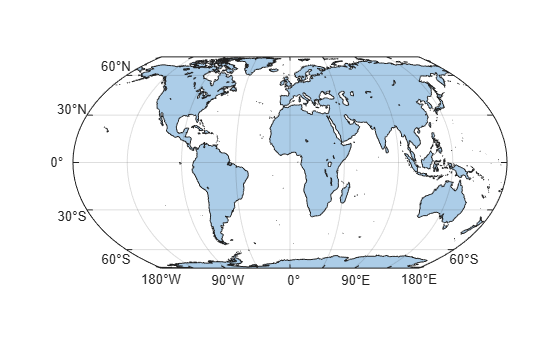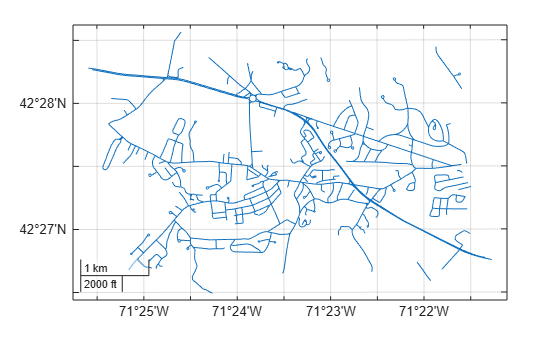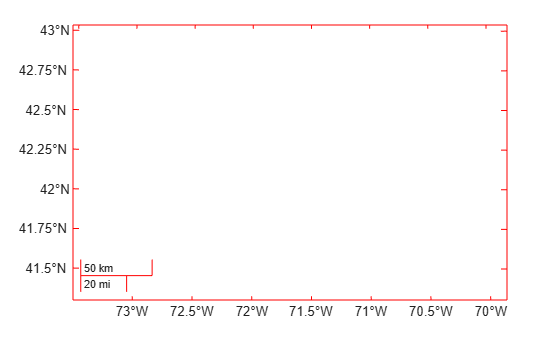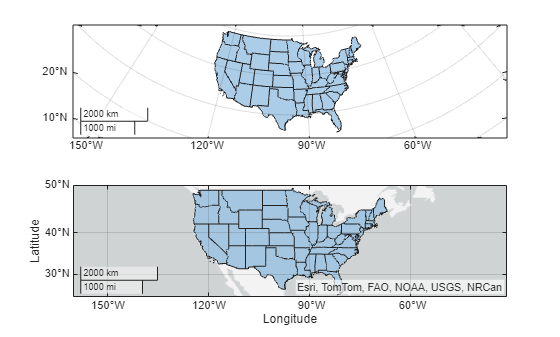# newmap

Set up new map

Since R2023a

## Syntax

``newmap``
``newmap(proj)``
``newmap(target,___)``
``mx = newmap(___)``

## Description

example

````newmap` creates the default map axes in the current figure. By default, the function creates a world map that uses an Equal Earth projection.In some cases, the `newmap` function can change the current figure and current axes. For more information, see Algorithms.```

example

````newmap(proj)` specifies the projected coordinate reference system (CRS) for the map.```
````newmap(target,___)` changes or replaces the axes specified by `target` instead of the current axes, in addition to any combination of inputs from the previous syntaxes.```

example

````mx = newmap(___)` returns the `MapAxes` object. Use `mx` to query and modify properties of the `MapAxes` object after creation. For a list of properties, see MapAxes Properties.```

## Examples

collapse all

Create the default map axes. By default, the map axes uses an Equal Earth projection.

`newmap`

Read a shapefile containing world land areas into the workspace as a geospatial table. Display the land areas on the map axes.

```land = readgeotable("landareas.shp"); geoplot(land)````MapAxes` objects use projected coordinate reference systems (CRSs) to transform geographic (latitude-longitude) coordinates to projected (xy) coordinates. There are two ways to get a projected CRS:

• Get a projected CRS from a data file.

• Create a projected CRS object by using the `projcrs` function. You can create a projected CRS object from an authority code or from a well-known text string.

Get Projected CRS from File

Read a shapefile of road data into the workspace as a geospatial table. The table represents the roads using line shapes in projected coordinates.

`roads = readgeotable("concord_roads.shp");`

Extract the projected CRS from the data file. Then, create a map axes that uses the projected CRS.

```proj1 = roads.Shape.ProjectedCRS; newmap(proj1)```

Display the data on the map axes.

`geoplot(roads)`Get Projected CRS from Authority Code

Read a shapefile of US states into the workspace as a geospatial table. The table represents the states using polygon shapes in geographic coordinates. Extract the table row representing Alaska.

```states = readgeotable("usastatehi.shp"); alaska = states(states.Name == "Alaska",:);```

Create a projected CRS object appropriate for Alaska. Use the Alaska Albers Equal Area Conic projected CRS, which has the ESRI code `102006`. Then, create a map axes that uses the projected CRS.

```proj2 = projcrs(102006,Authority="ESRI"); newmap(proj2)```

Display the Alaska polygon on the map axes.

```geoplot(alaska) geolimits([48 72],[-180 -135])```Import a shapefile containing road data for Concord, MA into the workspace as a geospatial table. The table represents roads using line shapes in projected coordinates.

`roads = readgeotable("concord_roads.shp");`

Create a map axes that uses the projected CRS embedded in the data file. Return the `MapAxes` object as a variable.

```proj = roads.Shape.ProjectedCRS; mx = newmap(proj);```Customize the map by setting properties of the `MapAxes` object. Set the tick label format to decimal degrees, remove the graticule lines, and change the outline color.

```mx.TickLabelFormat = "dd"; mx.GraticuleLineStyle = "none"; mx.OutlineColor = "r";```Read a shapefile of US states into the workspace as a geospatial table. The table represents the states using polygon shapes in geographic coordinates. Remove the table rows representing Alaska and Hawaii.

```states = readgeotable("usastatehi.shp"); idx = states.Name ~= "Alaska" & states.Name ~= "Hawaii"; states = states(idx,:);```

Create a 2-by-1 tiled chart layout.

• In the first tile, display the US states over a map axes that uses a North America Albers Equal Area Conic projection.

• In the second tile, display the US states over a geographic axes, which uses a Web Mercator projection.

```tiledlayout(2,1) nexttile proj = projcrs(102008,Authority="ESRI"); newmap(proj) geoplot(states) nexttile geoplot(states)```## Input Arguments

collapse all

Projected coordinate reference system (CRS), specified as a `projcrs` object. `MapAxes` objects use the projection method and projection parameters stored in the projected CRS to transform geographic (latitude-longitude) coordinates to projected (xy) coordinates.

By default, map axes objects use the World Geodetic System of 1984 (WGS 84) / Equal Earth Greenwich projected CRS, which has the EPSG code `8857`.

The projected CRS must have a forward and inverse projection implementation. Most projected CRSs have a forward and inverse projection implementation.

Target to change or replace, specified as one of these options:

• An axes object — An `Axes`, `PolarAxes`, `GeographicAxes`, or `MapAxes` object.

• A parent container — A `Figure`, `Panel`, `Tab`, `TiledChartLayout`, or `GridLayout` object.

If you do not specify this argument, then the `newmap` function uses the current axes.

## Algorithms

The behavior of the `newmap` function depends on the `NextPlot` properties of the figure and axes.

First, the `newmap` function queries the `NextPlot` property of the figure.

• If you specify the `target` input argument, then the function uses the figure associated with `target`.

• If you do not specify the `target` input argument, then the function uses the current figure. If there is no current figure, then the function creates a new figure.

This table shows how the `newmap` function changes the figure based on the value of `NextPlot`.

Value of `NextPlot`

Behavior of `newmap`

`"add"` (default)

The `newmap` function:

• Uses the current figure or the figure associated with `target`

• Does not remove child objects from the figure

• Does not reset figure properties

`"new"`

If you do not specify `target`, then the `newmap` function creates a new figure and uses it as the current figure.

If you specify `target`, then the function uses the figure associated with `target`.

`"replacechildren"`

The `newmap` function:

• Uses the current figure or the figure associated with `target`

• Removes all child objects, which are not hidden, from the figure

• Resets the `NextPlot` property of the figure to `"add"`

This behavior is similar to using the `clf` function.

`"replace"`

The `newmap` function:

This behavior is similar to using the `clf reset` syntax of the `clf` function.

Then, the `newmap` function queries the `NextPlot` property of the axes in the figure.

• If you specify the `target` input argument as an axes, then the function uses `target`.

• If you do not specify the `target` input argument, then the function uses the current axes. If there is no current axes, then the function creates a new `MapAxes` object.

This table shows how the `newmap` function changes the axes based on the value of `NextPlot`.

Value of `NextPlot`

Behavior of `newmap`

`"replace"` (default)

When the axes is a `MapAxes` object, the `newmap` function:

• Uses the specified axes

• Removes all child objects from the axes

• Resets axes properties, except `ProjectedCRS`, `Position` and `Units`, to their default values

When the axes is not a `MapAxes` object, the `newmap` function deletes the specified axes and creates a `MapAxes` object.

This behavior is similar to using the `cla reset` syntax of the `cla` function.

`"add"`

When the axes is a `MapAxes` object, the `newmap` function:

• Uses the specified axes

• Does not remove child objects from the axes

• Does not reset axes properties

When the axes is not a `MapAxes` object, the `newmap` function returns an error.

`"replacechildren"`

When the axes is a `MapAxes` object, the `newmap` function:

• Uses the specified axes

• Removes all child objects from the axes

• Resets the `ColorOrderIndex` and `LineStyleOrderIndex` properties of the axes to `1`, but does not reset other axes properties

When the axes is not a `MapAxes` object, the `newmap` function deletes the specified axes and creates a `MapAxes` object.

This behavior is similar to using the `cla` function.

`"replaceall"`

When the axes is a `MapAxes` object, the `newmap` function:

• Uses the specified axes

• Removes all child objects from the axes

• Resets axes properties, except `Position` and `Units`, to their default values

When the axes is not a `MapAxes` object, the `newmap` function deletes the specified axes and creates a `MapAxes` object.

This behavior is similar to using the `cla reset` syntax of the `cla` function.

## Version History

Introduced in R2023a